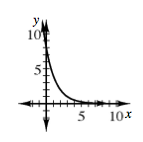### Home > CCA > Chapter 10 > Lesson 10.2.6 > Problem10-94

10-94.

An exponential function of the form $f(x)=ab^x$ passes through the points $(2,2)$ and $\left(4,\frac{1}{2}\right)$. .

1. Find the equation of the function.

Follow the steps shown in problem 10-33.

1. Use the equation to sketch a graph of the function.